Function Repository Resource:

# ParabolaProperties

Get an association of the properties of the given parabola

Contributed by: Wolfram|Alpha Math Team
 ResourceFunction["ParabolaProperties"][expr,{x,y}] returns an Association of properties of the parabola defined by expr.

## Details and Options

expr can be an equation or an Association of properties.
Parabola properties returned are "Equation", "Focus ", "Vertex", "Directrix", "FocalParameter", "SemiAxisLength", "AxisOfSymmetry", "SemiLatusRectum", "RotationAngle" and "BaseConic".
The "BaseConic" property is an association of the properties "Equation", "RotationAngle" and "DisplacementFromOrigin", where "Equation" represents an isomorphic conic section based at the origin and aligned with the x axis.
If the given expr describes a conic section other than a parabola, ResourceFunction["ParabolaProperties"] will issue a message indicating it is not a parabola, but will still return the association of properties pertaining to the correct conic section.

## Examples

### Basic Examples (1)

Compute the properties of a basic parabola:

 In:=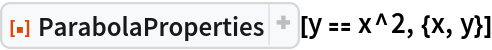Out=### Scope (2)

Compute the properties of a parabola described using other properties:

 In:=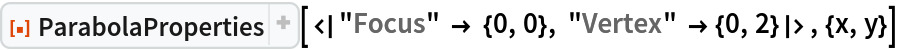Out=Compute the properties of a skew parabola (not aligned with the x or y axis) described by some properties:

 In:=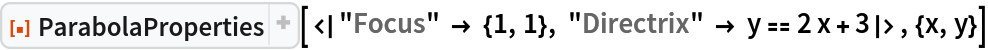Out=### Options (1)

If the input describes a well-defined parabola, ParabolaProperties defaults to not returning the "Type" property unless you specify it:

 In:=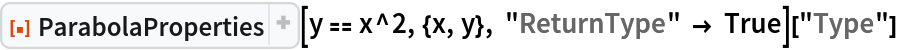Out=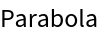### Properties and Relations (1)

Use the resource function InteractiveConicPlot to visualize conic properties:

 In:=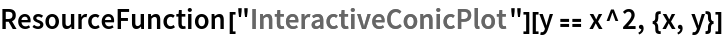Out=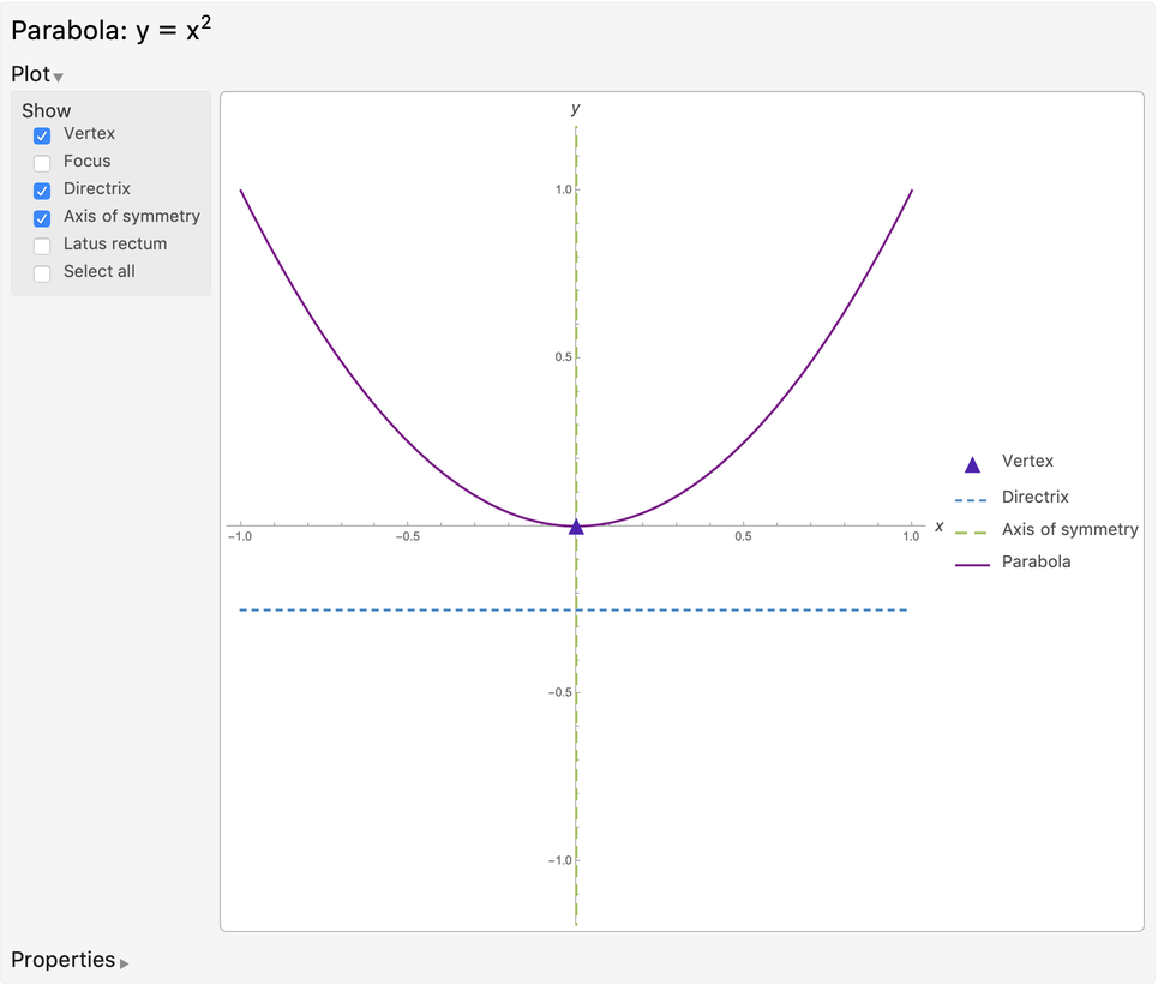### Possible Issues (1)

If the input equation or association does not describe a parabola but does describe another conic section, ParabolaProperties will return that instead, including the property "Type":

 In:=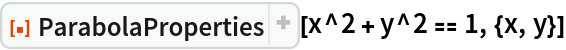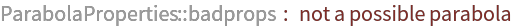Out=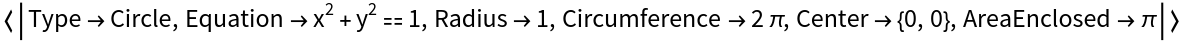## Publisher

Wolfram|Alpha Math Team

## Version History

• 3.0.0 – 23 March 2023
• 2.0.0 – 29 October 2020
• 1.0.0 – 09 April 2020